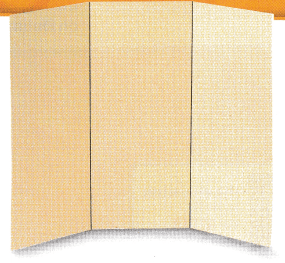# Texas Go Math Grade 6 Module 18 Answer Key Becoming a Knowledgeable Consumer and Investor

Refer to our Texas Go Math Grade 6 Answer Key Pdf to score good marks in the exams. Test yourself by practicing the problems from Texas Go Math Grade 6 Module 18 Answer Key Becoming a Knowledgeable Consumer and Investor.

## Texas Go Math Grade 6 Module 18 Answer Key Becoming a Knowledgeable Consumer and Investor

Write the fraction as a decimal and a percent.

Question 1.
$$\frac{1}{4}$$ ___________
Write the fraction as a division problem and affix zeroes on the dividend if necessary.0.25 × 100 = 25% multiply the decimal by 100 to make it in percent

Question 2.
$$\frac{4}{5}$$ ___________
Write the fraction as a division problem and affix zeroes on the dividend if necessary.0.8 × 100 = 80% multiply the decimal by 100 to make it in percent.

Question 3.
$$\frac{1}{10}$$ ___________
Write the fraction as a division problem and affix zeroes on the dividend if necessary.0.1 × 100 = 10% multiply the decimal by 100 to make it in percent.

Question 4.
$$\frac{5}{8}$$ ___________
Write the fraction as a division problem and affix zeroes on the dividend if necessary.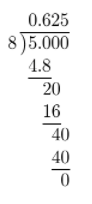0.625 × 100 = 62.5% multiply the decimal by 100 to make it in percent.

Find the sum.

Question 5.
4.9 + 26.78
Determine the sum of the decimal numbers. Align the decimal points and affix zeros if necessary.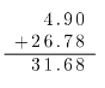The sum is 31.68.

Question 6.
3 + 13.792
Determine the sum of the decimal numbers. Align the decimal points and affix zeros if necessary.The sum is 16.792.

Question 7.
65.8 + 88.39
Determine the sum of the decimal numbers. Align the decimal points and affix zeros if necessary.The sum is 154.19.

Question 8.
2.789 + 58.3
Determine the sum of the decimal numbers. Align the decimal points and affix zeros if necessary.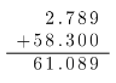The sum is 61.089.

Find the percent.

Question 9.
20% of 50 __________
Determine the percent of a number. First write the percent as a decimal number then multiply.
20% = 0.20Question 10.
8% of 72 __________
Determine the percent of a number. First write the percent as a decimal number then multiply.
8% = 0.08Question 11.
35% of 240 ___________
Determine the percent of a number. First write the percent as a decimal number then multiply.
35% = 0.35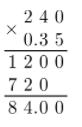Question 12.
14% of 18 __________
Determine the percent of a number. First write the percent as a decimal number then multiply.
14% = 0.14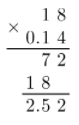Question 13.
145% of 80 ________
Determine the percent of a number. First write the percent as a decimal number then multiply.
145% = 1.45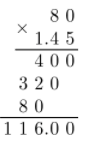Question 14.
4.3% of 700 __________
Determine the percent of a number. First write the percent as a decimal number then multiply.
4.3% = 0.043Visualize Vocabulary

Use the ✓ words to complete the graphic.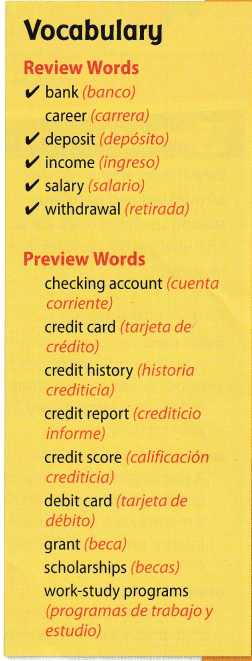Understand Vocabulary

Complete the sentences using the preview words.

Question 1.
When you use a ___________, the money you spend is deducted immediately from your checking or savings account.
When you use a ___________, you pay for your purchases later.
The missing information that will complete the sentences are debit card and credit card.

Question 2.
A ____________ includes information about how well you manage money.